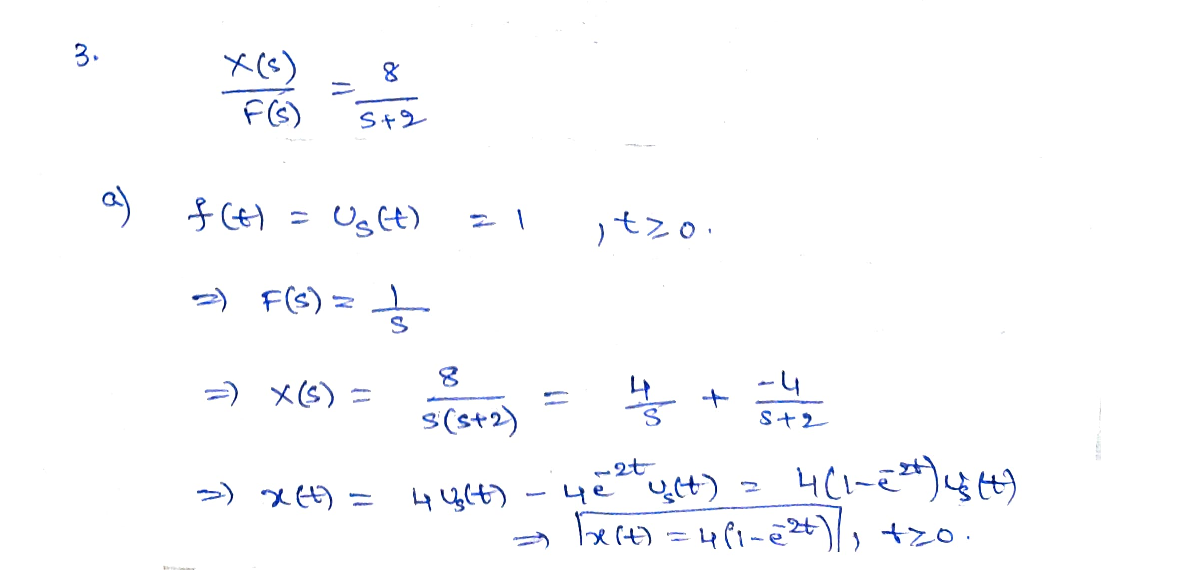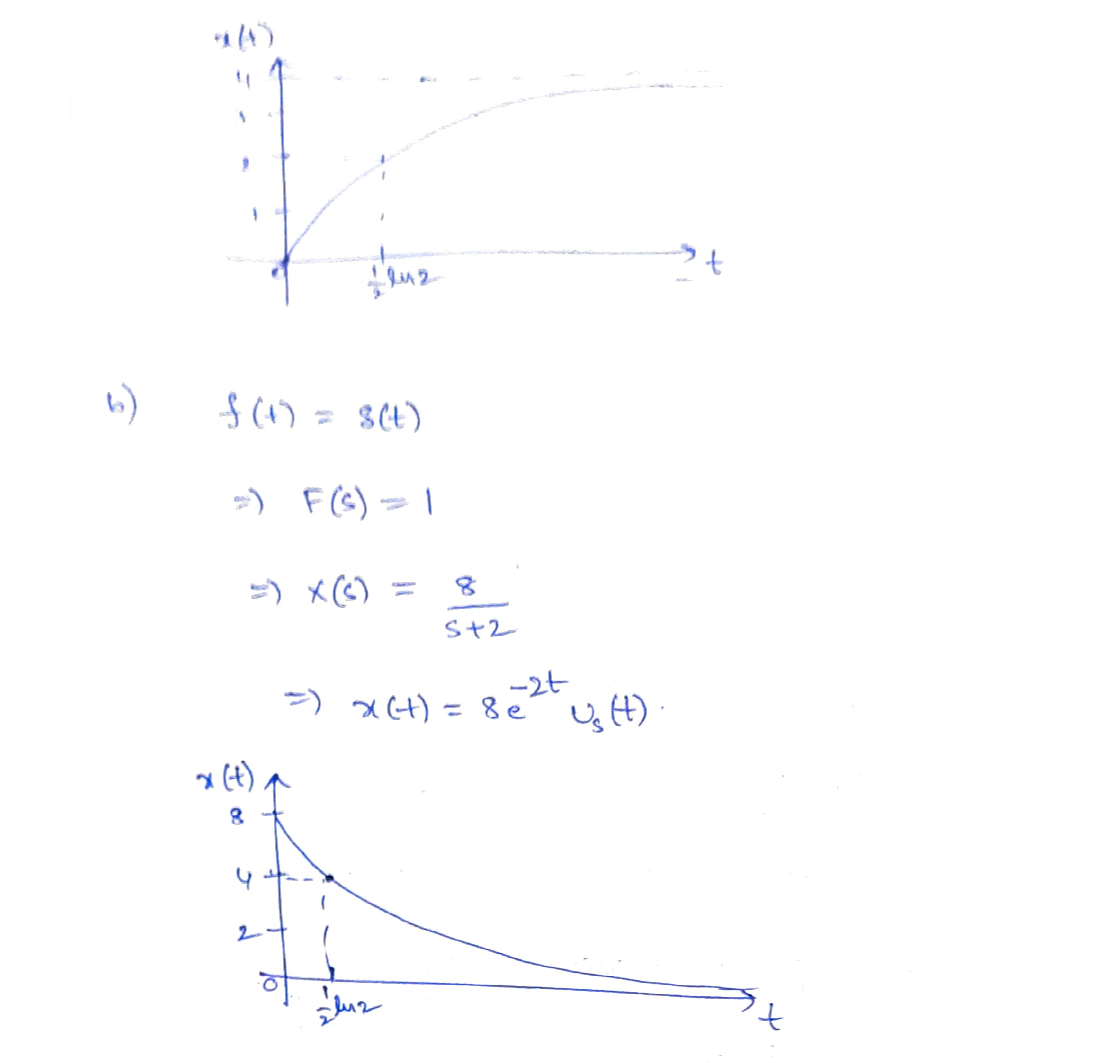Question

System Dynamics

3. Consider a system that has a transfer function

\frac{X(s)}{F(s)}=\frac{8}{s+2}

Find the response x(t) when the input f(t) is

a unit step function u(t)=1 for t >= 0, and

an impulse (Dirac delta) function 8(t) occurring at time t = 0.Verified### Question 36905System Dynamics

10.4 Figure Pl0.4 shows a closed-loop control system.
a. Compute the controller gain Kp so that the undamped natural frequency of the closed-loop system is w, = 4 rad/s.
b. Compute the controller gain Kp so that the damping ratio of the closed-loop system is = 0.7.
Compute the steady-state output for a step reference input r(t)=4u(t) and controller gain kr=2

### Question 36904System Dynamics

Figure P10.3 shows a general closed-loop control system. The plant transfer function is
a. Determine whether the closed-loop system is stable for control gain K, 2.
b. Compute the controller gain Kp so that step response shows 25% overshoot.
c. Estimate the settling time for a step reference input if the control gain is K, 0.5.
G_{p}(s)=\frac{1}{s^{2}+6 s+8}

### Question 36903System Dynamics

IFigure P10.1 shows a general feedback control system with forward-path transfer functions Ge(s) (controller) and Gp(s) (plant) and feedback transfer functions H(s). Given the following transfer functions,determine the closed-loop transfer function T(s) = Y(s)/R(s).

### Question 35645System Dynamics

Problem 5. (5 points) Consider the dynamic system that has negative real poles only.Determine the transfer function from the asymptote of the Bode magnitude plot shown below:

### Question 35644System Dynamics

Problem 4. The closed-loop system is shown below. WVe want to draw its root-locus and design the positive constant K to achieve closed-loop stability.
Find the departure angles at pi and p2, and the arrival angle at 21.
\text { Departure angle: } \phi_{d e p}=\sum_{j=1}^{m} \psi_{j}-\sum_{i \neq d e p}^{n} \phi_{i}-180(2 k+1)
\text { Arrival angle: } \psi_{a r r}=\sum_{i=1} \dot{\phi}_{i}-\sum_{j \neq a r r}^{m} \psi_{j}+180(2 k+1)
(2) (2.5 points) Find the range of K for closed-loop stability using the Routh stability criterion.

### Question 35643System Dynamics

(2) (2.5 points) Determine K, and K2 such that wn = 4 rad/sec, and t, = 1 sec. Note:uhere u and t are the natural freguency and damning ratio respectivel:
whcre K1 and K2 arc the positive constants.
Derive the closed-loop sensitivity function: S(s) = E(s)/R(e).
Consider the closed-loop control system shown below:
t_{s}=\frac{4}{\omega_{n}} \text {, where } \omega_{n} \text { and } \zeta \text { are the natural frequency and damping ratio, respectively. }

### Question 35642System Dynamics

Problem 2. (1) (2.5 points) DErive the Equations of motion of a quarter-car model shown below. (2) (2.5 points) Obtain the state-space model. (3) (2.5 points) Obtain the transfer function: G(s) = Y(s)/R(s).

### Question 35641System Dynamics

1. (2.5 points) Solve the following ODE using the Laplace Transform approach.
\ddot{y}(t)+7 \dot{y}(t)+10 y(t)=4
y(0)=\dot{y}(t)=0
\text { Note: } \mathcal{L}(1)=\frac{1}{s} \text { and } \mathcal{L}\left(e^{-a t}\right)=\frac{1}{s+a} \text {. }

### Question 34038System Dynamics

a. Obtain the 1/O equation for this system where y is the output and u is the input.
a. Obtain the 1/O equation for this system where y is the output and u is the input.
\dot{x}=\left[\begin{array}{cc} 0 & 1 \\ -20 & -4 \end{array}\right] x+\left[\begin{array}{c} 0 \\ 0.2 \end{array}\right] u \quad y=\left[\begin{array}{ll} 1 & 0 \end{array}\right] x
b. Obtain the transfer function for this system.

### Question 34037System Dynamics

Given the following system equation
4 z+20 z+84 z=0.12 u
b. Derive the system transfer function G(s) = Z(s)/U(s)
a. Obtain a complete SSR with input u and output y 2.
Derive the transfer function Y(s)/U(s) where the output is y = ż.C.

### Submit query

Getting answers to your urgent problems is simple. Submit your query in the given box and get answers Instantly.

### Submit a new QuerySuccess

Assignment is successfully created